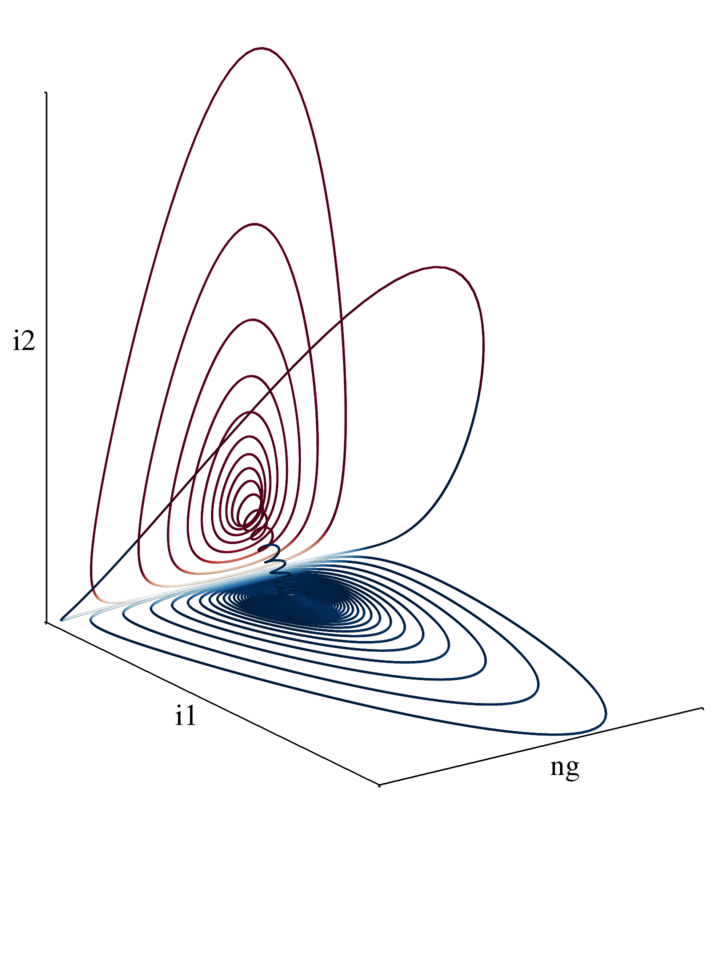```from mayavi import mlab
import pandas as pd
import numpy as np

colors = np.zeros_like(i1)

for i in xrange(colors.size):
colors[i] = abs(i1[i] + i2[i])
if i1[i] < i2[i]:
colors[i] = colors[i]*-1

mlab.options.offscreen = True

fig = mlab.figure(size=(1800,2800),bgcolor=(1,1,1))
fig.scene.parallel_projection = True

ax = mlab.axes( color=(0,0,0))
ax.property.line_width = 3.
ax.axes.label_format = ''
ax.axes.x_label= ''
ax.axes.y_label= ''
ax.axes.z_label= ''

mlab.draw()
mlab.view( 55, 70, focalpoint='auto', distance='auto' )

mlab.savefig('mayavi_phase_space.png')
```

#### titles, axis lables, sizes etc

mostly inspired by this discussion.. here, Gael suggests using inkscape to add labels and stuff.. this is definitely a potential approach, however, if you'd like to be able to easily reproduce the plot in the future without having to mess around in a GUI, ImageMagick is a great choice..

so, to chop off the edges of the plot..

```~/ \$ convert mayavi_phase_space.png -gravity North -chop 0x600 -gravity South -chop 0x250 \
-gravity East  -chop 200x0 -gravity West  -chop 150x0 chopped_phase_space.png
```

```~/ \$ convert chopped_phase_space.png -font Times-Roman -pointsize 60 -draw \